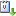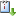Usted está aquí: Double Covers, Cancellation and Automorphisms.

# Double Covers, Cancellation and Automorphisms.

Ponente: Wilfried Imrich
Institución: Department Mathematics and Information Technology, Montanuniversität Leoben
Tipo de Evento: Investigación, Divulgación
Cuándo 10/09/2018 de 12:00 a 13:00 Sala A2 del Centro Académico Cultural (CAC)vCaliCal
The motivation for  this talk are the similarities of results  on automorphisms of double  covers  with those on the cancellation of graphs with respect to the direct product.  The \emph{double cover} of a graph $G$  is the direct product of $G$ by $K_2$, and $G$ is \emph{unstable} if the automorphism group of its double cover is larger than  $\operatorname{Aut}(G)\times \mathbb{Z}_2$. $G$ is called \emph{nontrivially unstable}, if it is non-bipartite and if any two distinct vertices have different neighborhoods, in other words, if $G$ is non-bipartite and thin.  1989 Maru\v{s}i\v{c}, Scapellato and Zagaglia Salvi proved  that to any nontrivially unstable graph $G$ there always exists a permutation matrix $P$ such that the product $AP$, where $A$ is the adjacency matrix of $G$, is the adjacency matrix of some graph. They asked when the converse holds.
Translated into the language of matrices  the answer of Mizzi and Scapellato form 2013 is that  $G$ is nontrivially unstable  if and only if there exists  a permutation matrix $P$ of order $>2$ such that $PAP=A$. We give a proof  using a result of Richard Hammack about antiautomorphisms and cancellation properties of direct products.
The talk also presents the background and discusses related results and problems about direct products.